﻿ 石墨烯增强复合材料截顶圆锥壳的自由振动 Free Vibration of Graphene Reinforced Composite Truncated Conical Shell

International Journal of Mechanics Research
Vol. 08  No. 02 ( 2019 ), Article ID: 30639 , 8 pages
10.12677/IJM.2019.82012

Free Vibration of Graphene Reinforced Composite Truncated Conical Shell

Yi’an Wang, Yanping Sheng*, Pengcheng Jiang

School of Applied Science, Beijing Information and Science Technology University, Beijing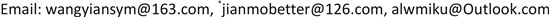Received: May 15th, 2019; accepted: May 29th, 2019; published: Jun. 5th, 2019ABSTRACT

Graphene reinforced composite is a new generation of advanced composite materials. In this material, graphene platelet as a reinforcement is randomly or evenly distributed in the matrix with gradient or uniform arrangement according to a certain rule along the thickness. The mechanical behavior of graphene-reinforced composites and their structures has become a hotspot in the research field in recent years. Based on energy conservation and Rayleigh-Ritz numerical method, the natural frequencies of free vibration of graphene reinforced composite truncated conical shells under simply supported boundary conditions are obtained. Further, parametric studies show fundamental frequency of graphene-reinforced compositetruncated conical shellincreases monotonously with the increase of graphene weight fraction as well as bottom radius-to-thickness ratios and is greatly influenced by the distribution pattern of graphene nanoplatelets. The dimensionless fundamental frequency increases monotonously first and then decreases monotonously with the semi-vertex angle.

Keywords:Graphene Reinforced Composite, Truncated Conical Shell, Free Vibration, Rayleigh-Ritz1. 引言

2. 石墨烯增强复合材料截顶圆锥壳及其物性参数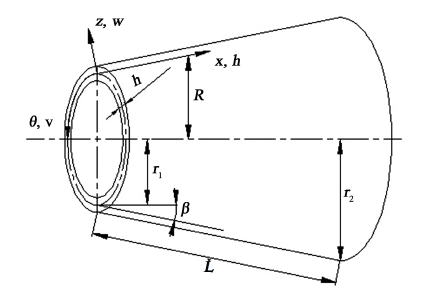Figure 1. Geometry of truncated conical shell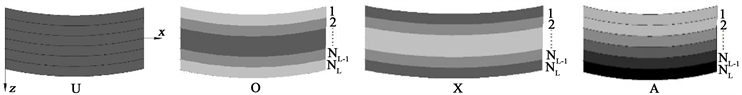Figure 2. Different graphene distribution patterns

${E}_{c}^{\left(k\right)}=\frac{3}{8}\frac{1+{\xi }_{1}{\eta }_{1}{V}_{G}^{\left(k\right)}}{1-{\eta }_{1}{V}_{G}^{\left(k\right)}}×{E}_{M}+\frac{5}{8}\frac{1+{\xi }_{2}{\eta }_{2}{V}_{G}^{\left(k\right)}}{1-{\eta }_{2}{V}_{G}^{\left(k\right)}}×{E}_{M},$ (1)

${\eta }_{1}=\frac{\left({E}_{G}/{E}_{M}\right)-1}{\left({E}_{G}/{E}_{M}\right)+{\xi }_{1}};\text{ }\text{ }\text{ }\text{ }\text{ }{\eta }_{2}=\frac{\left({E}_{G}/{E}_{M}\right)-1}{\left({E}_{G}/{E}_{M}\right)+{\xi }_{2}};\text{ }\text{ }\text{ }\text{ }{\xi }_{1}=\frac{2{l}_{G}}{{h}_{G}};\text{ }\text{ }\text{ }\text{ }{\xi }_{2}=\frac{2{w}_{G}}{{h}_{G}}.$ (2)

${E}_{M}$ , ${E}_{G}$ , ${l}_{G}$ , ${w}_{G}$${h}_{G}$ 分别为基体和石墨烯的杨氏模量、石墨烯小块的长度、宽度以及厚度，第k层石墨烯体积分数计算公式如下：

${V}_{G}^{\left(k\right)}=\frac{{w}_{G}^{\left(k\right)}}{{w}_{G}^{\left(k\right)}+\left({\rho }_{G}/{\rho }_{M}\right)\left(1-{w}_{G}^{\left(k\right)}\right)},$ (3)

${w}_{G}^{\left(k\right)}$ 是第k层石墨烯小块的质量分数，其在不同分布模式下的计算公式如下：

U： ${w}_{G}^{\left(k\right)}={f}_{G}.$ (4)

O： ${w}_{G}^{\left(k\right)}=4{f}_{G}\left(\frac{{N}_{L}+1}{2}-|k-\frac{{N}_{L}+1}{2}|\right)/\left(2+{N}_{L}\right).$ (5)

X： ${w}_{G}^{\left(k\right)}=4{f}_{G}\left(\frac{1}{2}+|k-\frac{{N}_{L}+1}{2}|\right)/\left(2+{N}_{L}\right).$ (6)

A： ${w}_{G}^{\left(k\right)}={f}_{G}\left(2k-1\right)/{N}_{L}.$ (7)

${\rho }_{c}^{\left(k\right)}={\rho }_{G}{V}_{G}^{\left(k\right)}+{\rho }_{M}\left(1-{V}_{G}^{\left(k\right)}\right);\text{\hspace{0.17em}}{v}_{c}^{\left(k\right)}={v}_{G}{V}_{G}^{\left(k\right)}+{v}_{M}\left(1-{V}_{G}^{\left(k\right)}\right).$ (8)

3. 位移场和本构关系方程

$\begin{array}{l}u\left(x,\theta ,z\right)={u}_{0}\left(x,\theta \right)+z{\phi }_{x}\left(x,\theta \right)\\ v\left(x,\theta ,z\right)={v}_{0}\left(x,\theta \right)+z{\phi }_{\theta }\left(x,\theta \right),\\ w={w}_{0}.\end{array}$ (9)

$\epsilon =AU.$ (10)

$A=\left(0\begin{array}{ccccc}{\partial }_{x}& 0& 0& z{\partial }_{x}& 0\\ 1/R\mathrm{sin}\beta & 1/R{\partial }_{\theta }& 1/R\mathrm{cos}\beta & z/R\mathrm{sin}\beta & z/R{\partial }_{\theta }\\ 1/R{\partial }_{\theta }& -1/R\mathrm{sin}\beta +{\partial }_{x}& 0& z/R{\partial }_{\theta }& z{\partial }_{x}-z/R\mathrm{sin}\beta \\ 0& -1/R\mathrm{cos}\beta & 1/R{\partial }_{\theta }& 0& 1\\ 0& 0& {\partial }_{x}& 1& 0\end{array}\right)$

${\partial }_{x},{\partial }_{\theta }$ 分别表示对空间坐标 $x,\theta$ 的偏导数。

${\sigma }^{\left(k\right)}={Q}^{\left(k\right)}\epsilon .$ (11)

${Q}^{\left(k\right)}=\left[\begin{array}{ccccc}{Q}_{{}_{11}}^{\left(k\right)}& {Q}_{{}_{12}}^{\left(k\right)}& & & \\ {Q}_{{}_{21}}^{\left(k\right)}& {Q}_{{}_{22}}^{\left(k\right)}& & & \\ & & {Q}_{{}_{44}}^{\left(k\right)}& & \\ & & & {Q}_{{}_{55}}^{\left(k\right)}& \\ & & & & {Q}_{{}_{66}}^{\left(k\right)}\end{array}\right]$ (12)

${Q}_{11}^{\left(k\right)}={Q}_{22}^{\left(k\right)}=\frac{{E}_{c}^{\left(k\right)}}{1-{\left({v}_{c}^{\left(k\right)}\right)}^{2}},{Q}_{11}^{\left(k\right)}={Q}_{21}^{\left(k\right)}=\frac{{v}_{c}^{\left(k\right)}{E}_{c}^{\left(k\right)}}{1-{\left({v}_{c}^{\left(k\right)}\right)}^{2}},{Q}_{44}^{\left(k\right)}={Q}_{55}^{\left(k\right)}={Q}_{66}^{\left(k\right)}=\frac{{E}_{c}^{\left(k\right)}}{2\left(1+{v}_{c}^{\left(k\right)}\right)}.$

4. 基于Rayleigh-Ritz方法固有频率的计算

$U=\underset{k=1}{\overset{{N}_{L}}{\sum }}{\int }_{{z}_{k}}^{{z}_{k+1}}{\int }_{0}^{L}{\int }_{0}^{2\text{π}}\left({\sigma }_{xx}^{\left(k\right)}{\epsilon }_{x}+{\sigma }_{\theta \theta }^{\left(k\right)}{\epsilon }_{\theta }+{\tau }_{x\theta }^{\left(k\right)}{\gamma }_{x\theta }+{\tau }_{xz}^{\left(k\right)}{\gamma }_{xz}+{\tau }_{\theta z}^{\left(k\right)}{\gamma }_{\theta z}\right)R\text{d}x\text{d}\theta \text{d}z,$ (13)

$T=\underset{k=1}{\overset{{N}_{L}}{\sum }}{\int }_{{z}_{k}}^{{z}_{k+1}}{\int }_{0}^{L}{\int }_{0}^{2\text{π}}{\rho }^{\left(k\right)}\left({\stackrel{˙}{u}}^{2}+{\stackrel{˙}{v}}^{2}+{\stackrel{˙}{w}}^{2}\right)R\text{d}x\text{d}\theta \text{d}z$ (14)

$x=0,x=L:{w}_{0}={v}_{0}={\phi }_{\theta }=0,$ (15)

$\left(\begin{array}{c}{u}_{0}\\ {v}_{0}\\ {w}_{0}\\ {\phi }_{x}\\ {\phi }_{\theta }\end{array}\right)=\underset{m=1}{\overset{M}{\sum }}\underset{n=1}{\overset{N}{\sum }}\left(\begin{array}{c}{u}_{mn}\mathrm{cos}m\theta \mathrm{cos}\frac{n\text{π}x}{L}\\ {v}_{mn}\mathrm{sin}m\theta \mathrm{sin}\frac{n\text{π}x}{L}\\ {w}_{mn}\mathrm{cos}m\theta \mathrm{sin}\frac{n\text{π}x}{L}\\ {\phi }_{xmn}\mathrm{cos}m\theta \mathrm{cos}\frac{n\text{π}x}{L}\\ {\phi }_{\theta mn}\mathrm{sin}m\theta \mathrm{sin}\frac{n\text{π}x}{L}\end{array}\right){\text{e}}^{i\omega t},$ (16)

$\begin{array}{l}\frac{\partial \left({T}_{\mathrm{max}}-{U}_{\mathrm{max}}\right)}{\partial {u}_{kj}}=0,\\ \frac{\partial \left({T}_{\mathrm{max}}-{U}_{\mathrm{max}}\right)}{\partial {v}_{kj}}=0,\\ \frac{\partial \left({T}_{\mathrm{max}}-{U}_{\mathrm{max}}\right)}{\partial {w}_{kj}}=0,\text{ }k=1\cdots ,M;j=1\cdots ,N.\\ \frac{\partial \left({T}_{\mathrm{max}}-{U}_{\mathrm{max}}\right)}{\partial {\phi }_{x}{}_{kj}}=0,\\ \frac{\partial \left({T}_{\mathrm{max}}-{U}_{\mathrm{max}}\right)}{\partial {\phi }_{\theta }{}_{kj}}=0.\end{array}$ (17)

$\left(K-{\omega }^{2}M\right)X=0.$ (18)

5. 数值算例

$\begin{array}{l}{\rho }_{G}=1.06\text{\hspace{0.17em}}\text{g}/{\text{cm}}^{\text{3}},{E}_{G}=1.01\text{\hspace{0.17em}}\text{TPa},{\nu }_{G}=0.186;{l}_{G}=2.5\text{\hspace{0.17em}}\mu \text{m},{w}_{G}=1.5\text{\hspace{0.17em}}\mu \text{m},{h}_{G}=1.5\text{\hspace{0.17em}}\text{nm},\\ {f}_{G}=1%,{\rho }_{M}=1.2\text{\hspace{0.17em}}\text{g}/{\text{cm}}^{\text{3}},{E}_{M}=3.0\text{\hspace{0.17em}}\text{GPa},{\nu }_{M}=0.34,L=0.8\text{\hspace{0.17em}}\text{m},h=0.002,{r}_{1}=0.5\text{\hspace{0.17em}}\text{m}.\end{array}$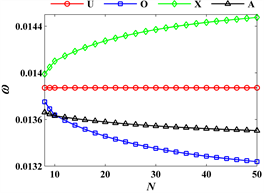Figure 3. Dimensionless fundamental frequency of truncated conical shells based on different total layers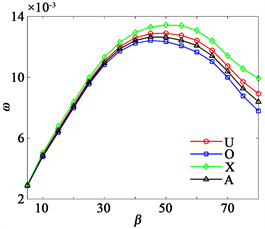Figure 4. Dimensionless fundamental frequency of truncated conical shells based on different semivertex angles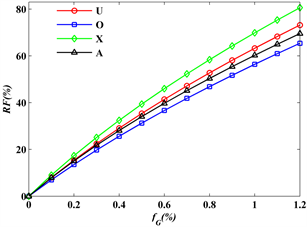Figure 5. Variation of dimensionless fundamental frequency of truncated conical shells with graphene weight fractionTable 1. The first six order dimensionless natural frequencies of system under different distributions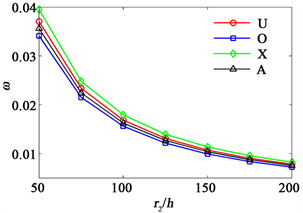Figure 6. Dimensionless fundamental frequency of truncated conical shells based on different bottom radius-to-thickness ratios

6. 小结

Free Vibration of Graphene Reinforced Composite Truncated Conical Shell[J]. 力学研究, 2019, 08(02): 101-108. https://doi.org/10.12677/IJM.2019.82012

1. 1. Rafiee, M.A., Rafiee, J., Yu, Z.Z. and Koratkar, N. (2009) Buckling Resistant Graphene Nanocomposites. Applied Physics Letters, 95, 223103. https://doi.org/10.1063/1.3269637

2. 2. Parashar, A. and Mertiny, P. (2012) Representative Volume Element to Estimate Buckling Behavior of Graphene/Polymer Nanocomposite. Nanoscale Research Letters, 7, 515. https://doi.org/10.1186/1556-276X-7-515

3. 3. Yang, J., Wu, H.L.and Kitipornchai, S. (2017) Buckling and Postbuckling of Func-tionally Graded Multilayer Graphene Platelet-Reinforced Composite Beams. Composite Structures, 161, 111-118. https://doi.org/10.1016/j.compstruct.2016.11.048

4. 4. Wang, A.W., Chen, H.Y., Hao Y.X. and Zhang, W. (2018) Vibration and Bending Behavior of Functionally Graded Nanocomposite Truncated Conical Shells Reinforced by Graphene Nanoplatelets. Results in Physics, 9, 550-559. https://doi.org/10.1016/j.rinp.2018.02.062

5. 5. Wang, Y.Q., Ye, C. and Zu, J.W. (2019) Nonlinear Vi-bration of Metal Foam Cylindrical Shells Reinforced with Graphene Platelets. Aerospace Science and Technology, 85, 359-370. https://doi.org/10.1016/j.ast.2018.12.022

6. 6. Guo, H.L., Cao, S.Q. and Yang, T.Z. (2018) Vibration of Laminated Composite Quadrilateral Plates Reinforced with Graphene Nanoplatelets Using the Element-Free IMLS-Ritz Method. International Journal of Mechanical Sciences, 142-143, 610-621. https://doi.org/10.1016/j.ijmecsci.2018.05.029

7. 7. Mao, J.J. and Zhang, W. (2018) Linear and Nonlinear Free and Forced Vibrations of Graphene Reinforced Piezoelectric Composite Plate under External Voltage Excitation. Composite Structures, 203, 551-565. https://doi.org/10.1016/j.compstruct.2018.06.076

8. 8. Reddy, J.N. (2004) Mechanics of Laminated Composite: Plates and Shells-Theory and Analysis.2nd Edition, CRC Press, Boca Raton. https://doi.org/10.1201/b12409

9. 9. Chen, H.Y., Wang, A.W., Hao, Y.X. and Zhang, W. (2017) Free Vibration of FGM Sand-wich Doubly-Curved Shallow Shell Based on a New Shear Deformation Theory with Stretching Effects. Composite Structures, 179, 50-60. https://doi.org/10.1016/j.compstruct.2017.07.032

10. 10. Yang, S.W., Hao, Y.X. and Zhang, W.(2015) Nonlinear Dynamic Behavior of Functionally Graded Truncated Conical Shell. International Journal of Bifurcation and Chaos, 25, 1550025. https://doi.org/10.1142/S021812741550025X

11. NOTES

*通讯作者。